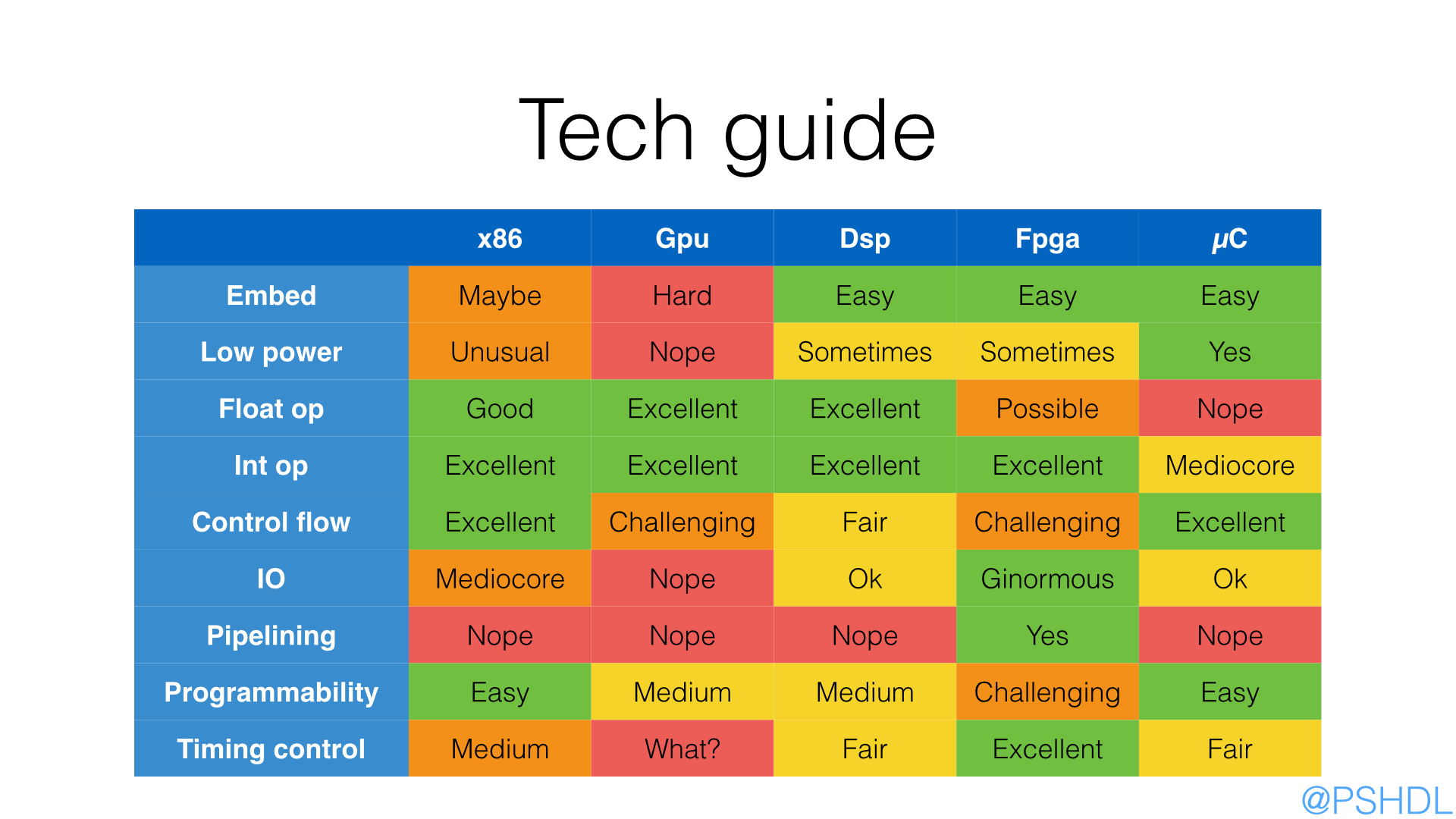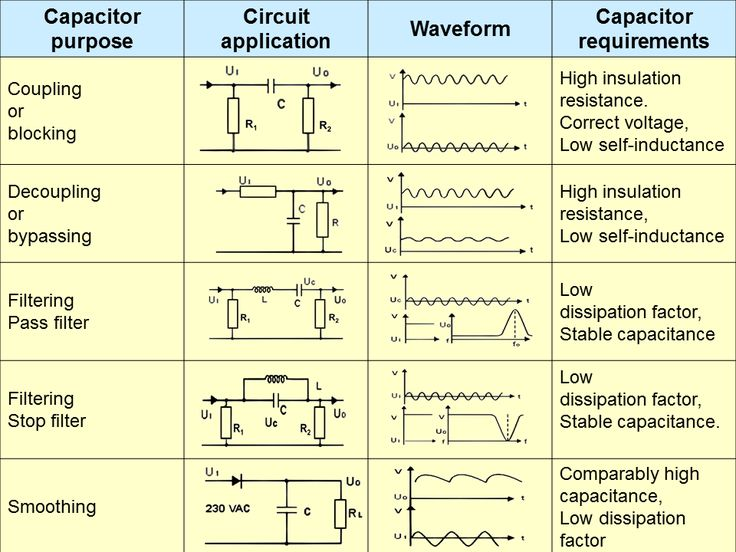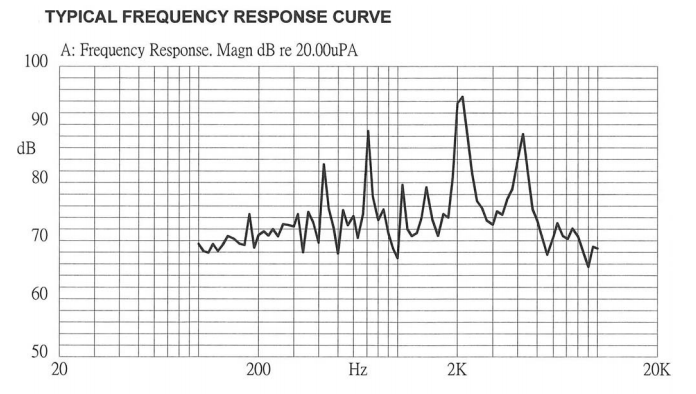## BASIC

The building blocks of every circuit are current and voltage: the first measures the number of electrons passing a section of conductor in unit of time, the unit of measure is the Ampere and it's indicated with $I$; the voltage is the difference of potential between two pieces of conductor and should generate a flow of current; its unit of measure is the Volt indicated with $V$.

The power consumption of a circuit is given by

.

• Site with pinout, tools etc: http://www.pighixxx.com/
• http://www.slideshare.net/balgan/hardware-hacking-101
• https://circuithub.com/
• http://blog.thelifeofkenneth.com/2013/02/diy-usb-power-strip.html
• http://123d.circuits.io/
• How do I read a datasheet?
• H Bridge Motor Speed Controller Tutorial
• Why 3.3v

## Cable&Wire

### AWG

This is the standard wire gauge system, increasing gauge numbers denote decreasing wire diameters; here the table from wikipedia.

• Wire Gauges The origin for the specification of the 'Gauge' of a wire is the number of dies that it has been pulled through - the higher the gauge, the more times it has been drawn.

### Crimping

• Video where is shown how to crimp manually a female header

### Magnetic (enameled) wire

It's the kind of wire used also for inductor coils.

### SMA connectors## Resistors

It's the basic component of electronics and it's indicated by the $R$ letter.

The unit is the Ohm and thanks to the previous formula we can link Ampere and Volt by the following relation

A particular type of resistence are the polyfuse that changes its value based on the current traversing it (first usage is overcurrent protection).

### Pull Up

• Pull-up Resistors
• http://www.resistorguide.com/pull-up-resistor_pull-down-resistor/

### Voltage divider

Be aware that this solution it's not ideal, you should use an NPN transistor in configuration emitter follower.

• Video from Afrotechmods

## Capacitors

This is another kind of component that is ancient: tipically consists of two plane near each other that accumulate electric charge.

The main formula is

The unit of capacitance is called Farad and has the following relation with the other units

There are different kind of capacitors, ceramic and electrolytic, each with pros and cons.

### Codes

The codes below are generally found on ceramic (little round discs) and mylar (chicklet) capacitors, which are not polarized, that is, there are no positive and negative leads (source).

Values Code
100pF 101
120pF 121
130pF 131
150pF 151
180pF 181
0.1uF 104
0.33uF 334

The general code is XXY, where

• XX is the coefficient
• Y is the esponent indicator $10^{-(11 - x)}$

Here a complete PDF with all the codes.

### Decoupling

A standard practice for electronic circuits is to add decoupling capacitors to reduce spikes and noise from power supplies and logic lines.

They have two main aims:

• charge supply
• filtering

The values of the capacitors depend on the frequencies of the AC components. Each capacitor has its own frequency response determined by its resistance and Equivalent Series Inductance (ESL) that is tuned to a range of frequencies. For example, to filter low frequencies you need a larger capacitor. As a rule, a capacitor of 0.1-1µF suffices for the mid-range frequencies, if you have slow fluctuations, you may choose around 1-10µF and for high-frequency noise you can use 0.001-0.1µF capacitors. You can also use any combination of bypass capacitors to remove a wider range of frequencies. For chips that drive a lot of current, you may put 10 µF - 100 µF capacitors to work as buffers. If the value of the capacitor allows, use monolithic ceramic capacitors because they are small and cheap.

• Capacitor decoupling: The act of (partially) separating the logic chip's power supply from the main power supply. In this video more explanation
• Choosing and Using Bypass Capacitors (PDF)
• Video: Decoupling capacitors and the 555 timer IC
• Video (and another video) with pretty good explanations of principle of decoupling.
• http://www.thebox.myzen.co.uk/Tutorial/De-coupling.html
• http://sites.fas.harvard.edu/~phys123/analog_notes/noise_note_sept09.pdf## Inductors

They are like capacitors, but for magnetic fields.

The unit of measure is the Henry composed by

• Magnetic core
• http://computer.howstuffworks.com/question352.htm

A radio-frequency choke (RFC) is used in the collector circuit to provide a high reactance (ideally open circuit) at the frequency of oscillation, ( ƒr ) and a low resistance at DC to help start the oscillations.

• https://e2e.ti.com/blogs_/b/analogwire/archive/2014/04/23/when-to-use-an-rf-choke-vs-an-inductor

## Impedance

Electrical impedance is the measure of the opposition that a circuit presents to a current when a voltage is applied.

For a DC circuit it coincides with the resistance, for an AC circuit is a complex number that takes into account the inductance and capacitance effects.

The analytical form for each type of components is the following:

## Switch

There are several codes related to this passive element (see wikipedia)

• N.O: normally open
• N.C: normally closed
• SPST: Single pole, single throw
• SPDT: Single pole, double throw

• Contatti digitali

## Buzzer

They are sound devices, activated by electromagnetic effects; they have some frequencies that are more loud than other, you have to see their response curve like this oneA code sample in an Arduino is

#define ALARM 8
#define SOUND_PARAMETER 300

void sound() {
for (int i = 0 ; i < SOUND_PARAMETER; i++) {
analogWrite(ALARM, 255);
delayMicroseconds(200);

analogWrite(ALARM, 0);
delayMicroseconds(200);
}

delay(SOUND_PARAMETER);
}


## Rotatory encoder

• http://www.hobbytronics.co.uk/tutorials-code/arduino-tutorials/arduino-tutorial6-rotary-encoder

## CMOY

• http://tangentsoft.net/audio/cmoy-tutorial/

## LCD

• http://jormungand.net/projects/devices/avrlcd/